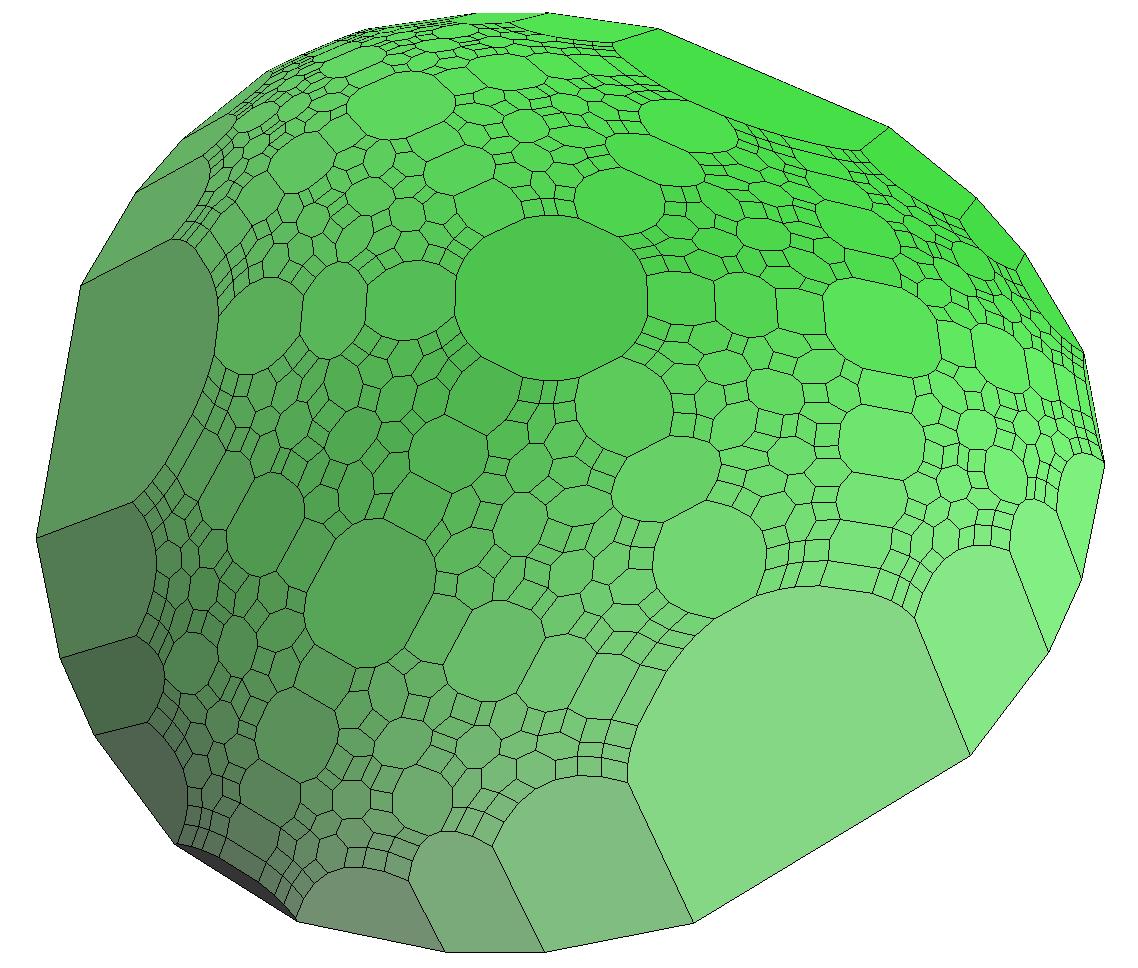# BENSOLVE

#### A Free Vector Linear Program Solver

BENSOLVE is a solver for vector linear programs (VLP), in particular, for the subclass of multiple objective linear programs (MOLP). It is based on Benson's algorithm and its extensions.

BENSOLVE is a free software published under the terms of the GNU General Public License. It utilizes the GNU Linear Programming Kit (GLPK). BENSOLVE (from version 2) is written in C programming language.

BENSOLVE provides the following features:

• arbitrary pointed solid polyhedral ordering cones
• primal and dual algorithms
• primal and dual solutions
• support of unbounded problems
• 3D graphics format output

Bensolve is used by the Octave / Matlab package BENSOLVE TOOLS, which is available at http://tools.bensolve.org. BENSOLVE TOOLS (but not BENSOLVE) provides many additional features, such as

• Calculus of Convex Polyhedra
• Calculus of Polyhedral Convex Functions
• Global Optimization